# Time Spread (Reverse Count from point in time)edited November 2022 in Modeling

Hi, I have a problem I am finding difficult to solve. Hopefully, someone in the community has encountered this before and can provide some clever solutions to this.

First of all, the modules I am showing here are just to explain the problem I am trying to solve. The actual modules are on a very large data set so I need to keep the line item count low.

I need to spread an amount (project cost) over time, based on a spreading rule and starting from a point in time, based on the project finish month.

#1 First of, I have this spreading table (below) which has just one line item to hold the percentage used to spread the project cost amount. On the columns, I have one list (Project time settings), where the "0" is the month of the project finish and "-1" is one month prior to the project finish month, "1" is one month after the project finish month, and so on.

In the rows I have one list (project time spread rules) which determines what pattern the amount spreading should follow and how much should be spread at each point in time.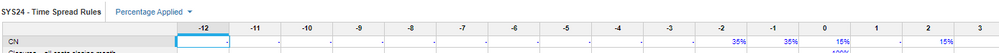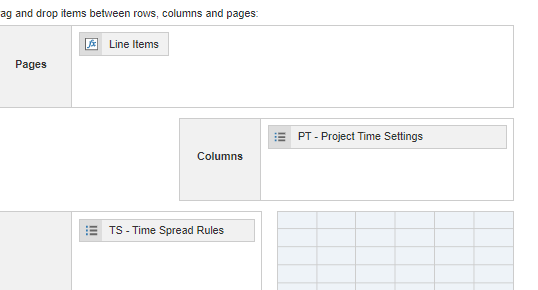#2 I also have the data module which contains one list (Accounts) and line items: project cost, Time spread rule (same list as list dimension in #1), and project finish month.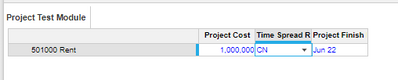#3 Finally, I have my calculation module which is essentially the same as the data module in #2, but with time (month) as a dimension as well. The rent account has 1 000 000 USD that should be spread with starting point (Jun 22), based on the spreading rule "CN". If we look at the spreading table in #1 that means in month 0 (Jun22), 15% of 1 000 000 should be allocated, month -1 35%, month - 2  35% and month +2 15%.

If you look at the picture below you can see the amount spread in the Project Cost Spreading. The thing that is not working right now and that I need help with is the logic for the line item "Time Spread Number". It should count the period forward and backward from the month of the project finish date. So in this example the project ended on Jun 22 which then becomes month 0, May 22 becomes -1 Jul 22 then becomes 1, and so it continues in both directions.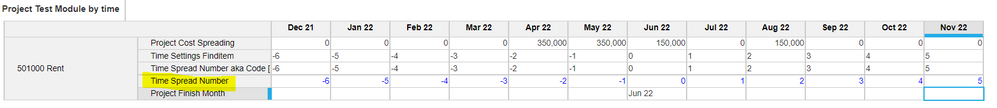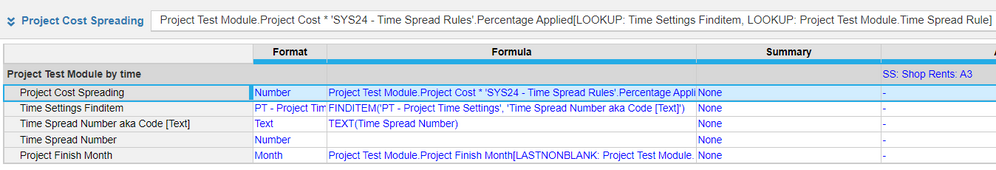Kind regards

Tagged:

•I have updated my solution.

• Create one line item in your SYS Time module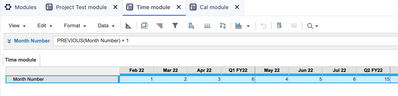• Project End, applies to Accounts only, direct reference from #2 module
• Current Time, applies to Time only, Formula :- Item(Time)
• Time Spread formula:- Time module.Month Number[LOOKUP: Current Time] - Time module.Month Number[LOOKUP: Project End]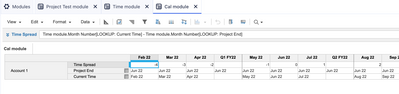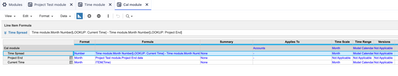I hope it solves your problem.

Thanks!

-Shubham

•edited November 2022

First of all, I'd like to appreciate the detailed explanation of your problem that you've written. Now, coming back to the solution. Sharing my idea below:-

• Add one list property to Project time settings list, name it sequence or number property (anything you like), number formatted.
• Input values corresponding to the list items 0 = 0, -1 = -1 and so on.
• Good thing is that you have already written the formula in Time Settings Finditem, so the correct values are flowing in that line item already.
• In your Time Spread Number line item write the formula:- 'Project time settings'.'Property(Number)'[LOOKUP: Time Settings Finditem]

It'll bring those number directly.

Here are some mockups screenshots.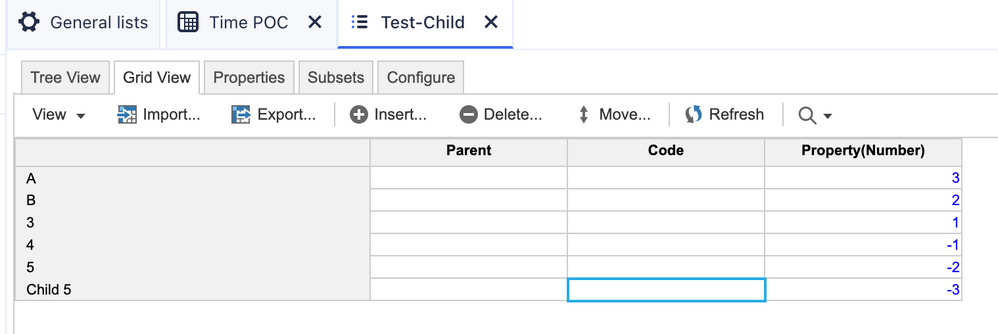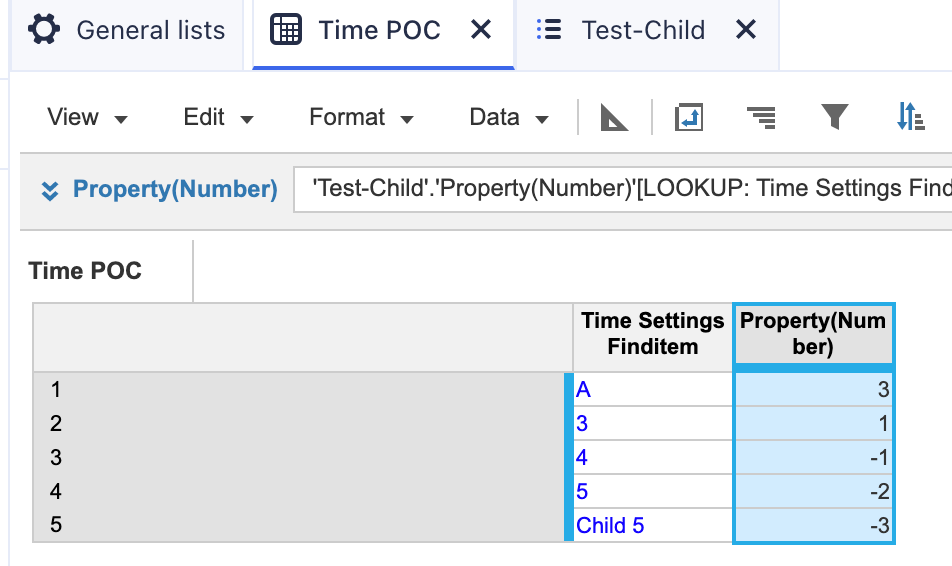I hope it solves your problem.

Thanks!

- Shubham

•edited November 2022

I think I need to clarify a little more. I need the line item "Time Spread Number" to give the correct number since that is the code that makes the Time Settings Finditem to work. The list members in the time settings list have the same code as their item name, so item 0 has code 0, item -1 has code -1, and so on. The reason why the finditem works are because I manually inputted the numbers in the "Time Spread Number" line item.

The logic flows Time Spread Number (Number format) ---> Time Spread Number aka Code (Text format) ---> Time Settings Finditem.

•edited November 2022

Let me know if the below solution works.

Data Module for current month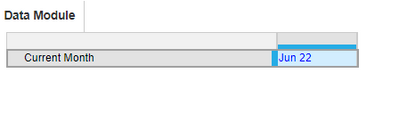Calculation Module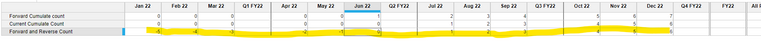Blue print view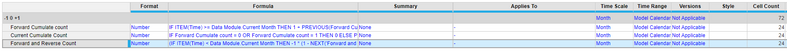Logics
IF ITEM(Time) >= Data Module.Current Month THEN 1 + PREVIOUS(Forward Cumulate count) ELSE 0
IF Forward Cumulate count = 0 OR Forward Cumulate count = 1 THEN 0 ELSE PREVIOUS(Forward Cumulate count)
(IF ITEM(Time) < Data Module.Current Month THEN -1 * (1 - NEXT('Forward and Reverse Count')) ELSE 0) + Current Cumulate Count

Thanks,
Manjunath

•edited November 2022

I see you have a list in applies to, since these calculations are independent of dimensions you need to remove applies to for these line items.

Thanks

•edited November 2022

Clearly, more simplified solution.

Cheers!

•edited November 2022

Thanks @ManjunathKN ! 😊

•Thank you! This was exactly what I needed.